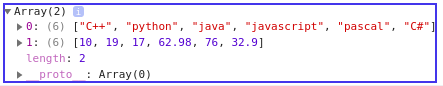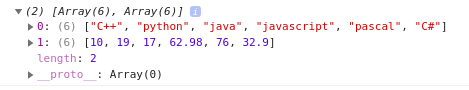Open in App
Not now

# How to call the map method only if the element is an array?

• Last Updated : 20 Dec, 2022

Given an object literal, let us say ‘person’. It has a number of properties of various types. The task here is to map the values of the property only if the property is an Array itself. Instead of an object, we can also perform the same set of operations on an array.

To achieve the above task, we can use the Array filter() method in JavaScript and then subsequently map the results.

The Array filter() method is used to create a new array from a given array consisting of only those elements which satisfy a condition set by the argument function.

The Array map() method in JavaScript creates an array by calling a specific function on each element present in the parent array.

Steps:

1. Convert the object values into an array using the Object.values() method. Let it be arr.
2. Iterate over the array(arr) using the filter method. The filter returns true if the type of the element is ‘object’.
3. Map the resulting array using the map method.

Following are the variations of the given problem:

Example 1:Mapping From an object literal the issue with the above-mentioned approach lies in the fact that neither the filter nor the map method can be used to iterate objects. To work around this problem, Object.Values should be used. Object.Values take an object as a parameter and return an array of all the values in that object. Now we can apply array map() and array filter() methods on this array.

```const person = {
first_name : 'John',
last_name : 'Doe',
skill_set : ['C++', 'python', 'java',
'javascript', 'pascal', 'C#' ],
fav_numbers : [10, 19, 17, 62.98, 76, 32.9],
email : 'john@someplace.com'
}

Output: (2) [Array(6), Array(6)]
0: (6) ["C++", "python", "java",
"javascript", "pascal", "C#"]
1: (6) [10, 19, 17, 62.98, 76, 32.9]```

Example:

## Javascript

 ``

Output:Console Output

Example 2: Mapping From an array, we can directly use array map() and array filter() methods here.

```const Person = ['John',8.6,['C++', 'python', 'java',
'javascript', 'pascal', 'C#' ],
'john@someplace.com',[10, 19, 17, 62.98, 76, 32.9]];

Output: (2) [Array(6), Array(6)]
0: (6) ["C++", "python", "java",
"javascript", "pascal", "C#"]
1: (6) [10, 19, 17, 62.98, 76, 32.9]```

Example:

## Javascript

 ``

Output:Console Output

My Personal Notes arrow_drop_up